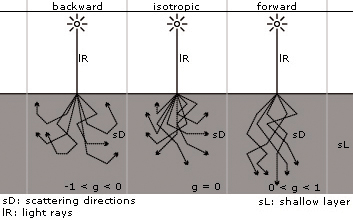### Optical Properties

The optical properties controlling the subsurface scattering shader are surface color, transmission color, refractive index, scattering and absorption coefficients, and the scattering function:

material
provides the material shader which determines the appearance of the material and the shading model to apply at a surface level.
transmission
is the transmission color or texture shader that filters light as it enters the object.
ior
is the index of refraction of the material, which determines how light changes direction as it enters or leaves the material.
absorption_coeff
is a measure of light attenuation as it passes through a turbid medium. Usually expressed in units of inverse length such that the product of the absorption coefficient and path length of a photon's travel through the medium is dimensionless. The probability of transmission is e-aL where a is the absorption coefficient and L is the path length. When using a coefficient that has been experimentally obtained, it is important to specify a correct scale conversion ratio between coefficient units and world units. Absorption is wavelength dependent.
scattering_coeff
is a measure of light scattering as it passes through a volume. This is usually expressed in units of inverse length so that the product of the scattering coefficient and path length of a photon's travel through the volume is dimensionless. The probability of transmission without redirection is e-sL where s is the scattering coefficient and L is the path length. When using a coefficient that has been experimentally obtained, it is important to specify a correct scale conversion ratio between the coefficient units and the world units. Scattering is wavelength dependent.
The table below gives suggested scattering and absorption coefficients (based on empirical data) for several materials.
Material Scattering_coeff Absorption_coeff ior
Jade 0.657 0.786 0.9 0.00053, 0.00123, 0.00213 1.3
Ketchup 0.18 0.07 0.03 0.061 0.97 1.45 1.3
Marble 2.19 2.62 3.00 0.0021 0.0041 0.0071 1.55
Skim milk 0.70 1.22 1.90 0.0014 0.0025 0.0142 1.3
Whole milk 2.55 3.21 3.77 0.0011 0.0024 0.014 1.3
scale_conversion
is a transform between the world coordinate system and the units used to represent the scattering and absorption coefficients. For example, if the world coordinate system is expressed in inches and the coefficients are in millimeters, then the correct scale factor is 25.4 (mm/inch).
scattering_anisotropy
is a measure of the degree of scattering. Specifically, it is the average dot product between the incident light direction and the scattered light direction. Most turbid materials do not scatter light uniformly. Values may range from -1 to 1. Where -1 corresponds to back scatter only and 1 corresponds to forward scatter only. The value 0 indicates uniform scattering in all directions.

The following simplified diagram traces the role of the scattering function:Schematic display of different values of g